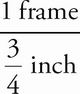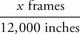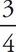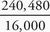# SAT Math Multiple Choice Question 221: Answer and Explanation

### Test Information

Question: 221

11. A single frame of 35 mm film is about three-quarters of an inch long. A film reel holds up to 1,000 feet of film. How many reels are required for a two-hour, forty-seven minute film shot at 24 frames per second?

• A. 13
• B. 14
• C. 15
• D. 16

D There are 12 inches in one foot, so a reel is 12 × 1,000 = 12,000 inches long. Set up a proportion to determine how many frames per reel:=. Cross-multiply to getx = 12,000. Divide both sides byto get x = 16,000 frames per reel. Next, find the number of frames the film requires. Convert the time to seconds. There are 60 minutes in an hour, so 2 hours and 47 minutes is equal to (2 × 60) + 47 = 167 minutes. There are 60 seconds in a minute, so there are 60 × 10,020 seconds in this film. If each second consists of 24 frames, then there are24 × 10,020 = 240,480 frames in this film. To determine the number of reels, divide by the number of frames per reel:= 15.03 reels. Because 15 reels does not hold quite enough frames, the film requires 16 reels, which is (D).